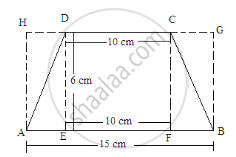Share

# Find the Area of a Trapezium Whose Parallel Sides of Lengths 10 Cm and 15 Cm Are at a Distance of 6 Cm from Each Other. Calculate this Area as The Sum of the Areas of Two Triangles and One Rectangle. - Mathematics

Course
ConceptArea of Trapezium

#### Question

Find the area of a trapezium whose parallel sides of lengths 10 cm and 15 cm are at a distance of 6 cm from each other. Calculate this area as
the sum of the areas of two triangles and one rectangle.

#### Solution

Given:
Length of the parallel sides of a trapezium are 10 cm and 15 cm.
The distance between them is 6 cm.
Let us extend the smaller side and then draw perpendiculars from the ends of both sides.Area of trapezium ABCD =(Area of rectangle EFCD)+(Area of triangle AED+Area of triangle BFC)
$=(10\times 6)+[(\frac{1}{2}\times AE\times ED)+(\frac{1}{2}\times BF\times FC)]$
$=60+[(\frac{1}{2}\times AE\times6)+(\frac{1}{2}\times BF\times6)]$
$=60+[3AE+3 BF]$
$=60+3\times(AE+BF)$
$\text{ Here, AE+EF+FB }= 15cm$
And EF = 10 cm
$\therefore AE+10+BF=15$
Or, AE+BF=15-10=5 cm
Putting this value in the above formula:
${\text{ Area of the trapezium }=60+3\times(5)=60+15=75 cm}^2$

Is there an error in this question or solution?

#### APPEARS IN

RD Sharma Solution for Mathematics for Class 8 by R D Sharma (2019-2020 Session) (2017 to Current)
Chapter 20: Mensuration - I (Area of a Trapezium and a Polygon)
Ex. 20.2 | Q: 7.1 | Page no. 22

#### Video TutorialsVIEW ALL 

Solution Find the Area of a Trapezium Whose Parallel Sides of Lengths 10 Cm and 15 Cm Are at a Distance of 6 Cm from Each Other. Calculate this Area as The Sum of the Areas of Two Triangles and One Rectangle. Concept: Area of Trapezium.
S